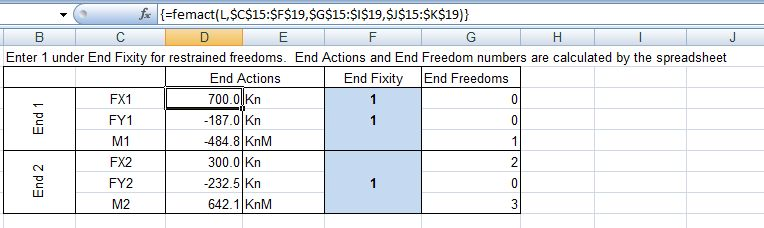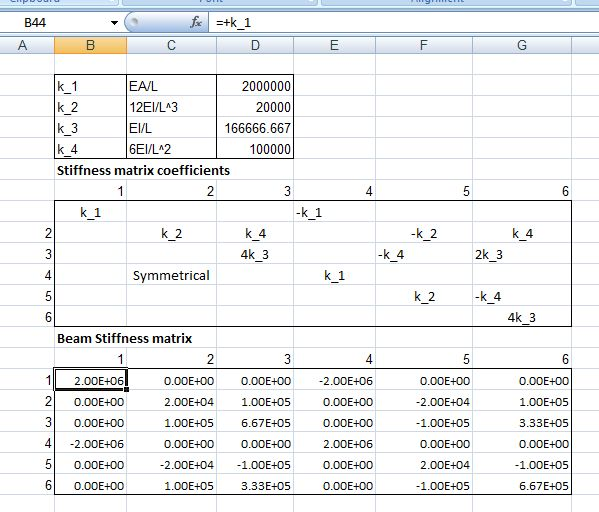## Frame Analysis with Excel 1 – Single beam

This is the first of a series of posts in which I will develop a structural frame analysis program operating within Excel.  The first few stages will be mostly spreadsheet based, with later stages using VBA routines to analyse data generated in the spreadsheet, and ultimately linking to external dll programs to do the heavy number crunching involved in the solution of complex frames.

This post will look at the analysis of a single 2D beam element subject to any combination of transverse and and axial loading, with any three of the six end freedoms restrained.  This will introduce the use of the stiffness method, setting up of a beam stiffness matrix and a matrix of end actions, and solution of these matrices using the Excel matrix functions.

Future posts will:

• Modify the beam analysis to deal with beams inclined to the global loading axes.
• Combine and solve the stiffness matrices for a number of straight continuous beams.
• Form and solve the stiffness matrix for a simple 2D frame.
• Convert the spreadsheet solution to a VBA routine suitable for solving large and complex 2D problems.
• Add provision for members with end moment or translational releases.
• Convert to 3D frames.
• Add provision for plotting of frame geometry, including applied loads, and plotting of analysis results.
• Convert the VBA analysis routines to a Fortran DLL, for improved performance.

As always, all the files presented here will be available for download, and all my code will be open source.  In addition to Internet resources, which will be referenced where applicable, the main sources of background information are:

The essence of the “stiffness” method of frame analysis is to set up a series of equations:

Kw = f

where K is a square matrix representing the “stiffness” of the frame, that is the deflection of the frame when subject to unit load; w is a column matrix representing the deflections of each node of the frame; and is a column matrix representing the loads applied to the nodes of the frame.

If K and f can be generated for any specified frame subject to a known set of loads, the deflections of each node can be found using the relationship:

 w =K-1 f

In this post the “frame” will consist of a single horizontal beam, and the matrices will be formed and solved on the spreadsheet, with the aid of a User Defined Function (UDF) to generate the applied nodal loads from the specified distributed and point loads and moments, that may be applied to any part of the beam.

The required input is shown in the screen shot below:

• Beam length
• Beam cross sectional area
• Beam second moment of area
• Beam Young’s Modulus
• Restrained end freedoms, three of vertical or horizontal deflection or rotation at either endThe nodal actions required for the analysis are the “fixed end” moments and reactions due to the applied loading, that is the end moments and reactions forces that would be generated by the applied loads if the ends of the beam were fully fixed against rotation and translation.  These are calculated by the UDF “FEMACT()”, which has as inputs the beam length, and the thre tables: distributed loads, point loads, and moments.  This UDF will be described in greater detail in a later post.  The output of the UDF is shown in the screen shot below:The formation of the beam stiffness matrix, and the matrix coefficients for the example beam are shown in the screen shot below.  The beam stiffness matrix coefficients are derived from the standard beam slope deflection equations, combined with the application of Hooke’s law for axial loads.  Further details of the derivation are given in many structural analysis textbooks.For the purposes of finding the magnitude of the unrestrained freedoms the beam stiffness matrix is reduced to 3×3 by extracting only the rows and columns related to the unrestrained freedoms, as shown in the screen shot below.  This matrix is then inverted, using the Excel Minverse function, then multiplied by the applied loads at the unrestrained nodes, using the Mmult function.  The result is a column of 3 cells giving the translations or rotations at the unrestrained nodes.  The final stage is to multiply the full original stiffness matrix by the full deflection column matrix to derive the nett force at each node.  The reactions at each node are then found by deducting the applied loads (i.e. the “fixed end” actions”).  It can be seen from the example output that the reactions at the unrestrained nodes are zero, and the reactions at the fixed nodes are equal and opposite to the applied loads, as would be expected.Inverted stiffness matrix and analysis results

This entry was posted in Excel, Finite Element Analysis, Frame Analysis, Newton, UDFs, Uncategorized and tagged , , , . Bookmark the permalink.

### 14 Responses to Frame Analysis with Excel 1 – Single beam

1.Tony says:

I was looking for a stiffness matrix for a two way slab action. This is the closest I could get. I tried to change the input apparently it didn’t work.

Thanx

Like

2.dougaj4 says:

Tony – the programs presented so far only deal with in-plane bending of a 2D frame. I will probably exytend it to 3D eventually, but that may be some way off.

Like

3.Ziad El Hachem says:

Hi I am very interested in your work, as I like to implement FEM in my excel sheet about micripile design. I work for a geotechnical firm in Lebanon, and I will study thorougly your sheets and try to implement them. I will stay in touch and talk about my work, and ask questions if needed.

Like

4.dougaj4 says:

Ziad – you might also find this post:

https://newtonexcelbach.wordpress.com/2009/07/05/continuous-beam-analysis-with-cubic-splines/

and the following related posts interesting.

If you are using these programs for real work please note the disclaimers and ensure that all results are properly verified.

Like

5.Ziad El Hachem says:

Hmm, I see your post, so what i am saying is:
i want to start from one of your program and add spring supports to it to model the soil. I used to be good with excel including VBA, but now i am concentration on using CALC instead. my question is: do you advice me to start from continuous beam or from frame model? also, can you suggest some reading or resource to implement spring supports? Thanks in advance.

Like

6.dougaj4 says:

The spreadsheet is set up to analyse laterally loaded piles. The only drawback at the momeny is that it only accepts linear elastic soil and concrete properties.

In the future I will be posting a version that alows for non linear soil (as in the old COM624 program) and also non-linear concrete, but I don’t know when.

Like

7.mambo says:

Hi,

in the Excel 1 – Single beam spreadsheet, in the applied load section;
1. numbers 1 to 5, are there number of loading conditions apply to the beam?
2. how should I derive the ‘position’ in the point load and moment section? What is ‘position’ means actually?

thanks.

Like

8.dougaj4 says:

Mambo – the spreadsheet only allows for 1 load condition, so all the specified loads are combined. If you want different load conditions you would need to enter them separately, and copy and paste the results for each condition.

The position of the point loads and point moments is the distance of the load from the left hand end of the beam. I should have said that.

For the distributed loads the colums are:
Distance of the left hand end of the load from the left hand end of the beam.

Like

9.marlon napster says:

hello… i have tried to use the program, the system works well

it’s quite awesome…

unfortunately I’ve noticed that error is encountered when it comes to solving propped beams?

Like

10.dougaj4 says:

This site uses Akismet to reduce spam. Learn how your comment data is processed.